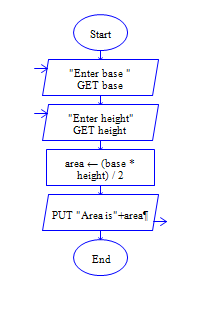# Area of triangle in Python

Python program to get base width and height of triangle and find area of triangle

Sample Input 1:

4 5

Sample Output 1:

10

#### Flow Chart Design#### Program or Solution

``` b=int(input("Enter the base:")) h=int(input("Enter the height:")) area=(b*h)/2 print("Area: {}".format(area)) ```

#### Program Explanation

get height and base of a triangle using input() method calculate area = height * base